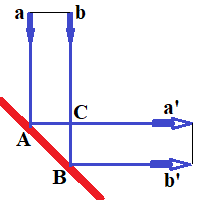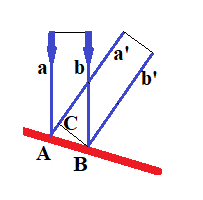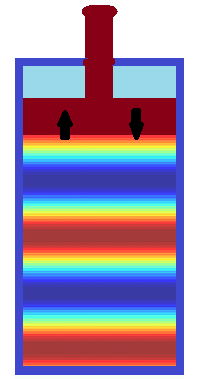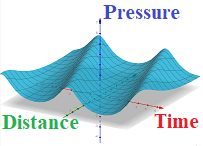## Saturday, August 7, 2021

### Reflection of Waves: UNIZOR.COM - Physics4Teens - Waves - Waves in Medium

Notes to a video lecture on http://www.unizor.com

Reflection Waves

Let's consider the same simple device as in the previous lecture - a cylinder of relatively small diameter with air and a piston in it, except this time we will examine what happens at the opposite to a piston end of a cylinder.Let's give a single short and quick push to a piston down. As a result, a single parallel to a piston wave of a higher pressure air will start its way down a cylinder with certain speed v that depends on properties of air, its temperature, initial pressure etc.

When this wave reaches the bottom of a cylinder, a thin layer of higher pressure air will develop there. Since the bottom of a cylinder prevents a wave to go farther, the higher pressure air will release its pressure back to a layer above it. This constitutes a reflection of the wave.

After such a reflection the wave will go to the opposite direction (upward on the picture) until it reaches the top, where piston is positioned. At this moment, since piston is not moving, it will play the same role as a bottom of a cylinder before, it will reflect the wave.

Ideally, this reciprocal movement of a wave can continue, it will be reflected from both ends of a cylinder indefinitely.
Practically, the energy of the initial push of a piston will gradually dissipate into more intense movement of the air molecules, which means that the temperature of the air will increase slightly.

Consider now a harmonic oscillation of a piston. It produces pressure waves in the air propagated downward. But now we know that they reflect from the bottom of a cylinder and propagate upwards.
This results in a very complex superposition of two sets of waves, one going downwards and another upwards.
There is, generally speaking, a shift in phase between these two sets of waves that depends on the speed of wave propagation and the length of a cylinder.

The resulting picture of air pressure will look quite chaotic and far from harmonic oscillations of a piston. Yet, it's just a simple superposition of waves moving in two opposite directions.

Imagine a complexity of a sound distribution in an opera house with air pressure waves, originated by a person singing on a stage, reflected from all hard surfaces and mixing in time and space with other waves. Unless special design measures are taken, the quality of a sound will be very low.

Even in such a simple case as recording these lectures we had to use special directional microphone that suppresses sounds from all directions except the main one directed onto a primary source of sound. In addition, to weaken the reflection, we open closets on the opposite wall to weaken the strength of reflected sound waves.

Let's analyze the reflection of a simple flat wave from a surface that is not perpendicular to the wave front, keeping in mind the Law of Reflection, that is, the angle of incidence equals to the angle of reflection.
Assume that our flat wave consists of only two molecules of air a and b that hit a surface AB at 45° angle.
A thin line between these molecules represents a wave's front, which is perpendicular to their trajectories.From a picture above and some elementary geometry it's obvious that reflected wave will be exactly as original, except its direction will be perpendicular to the original's one.
Indeed, a and b move on parallel trajectories with the same speed, covering the same distance in equal times. So, molecule a will come to point C at the same time as molecule b reaches point B. After that a and b continue their parallel movement and the new wave's front is still perpendicular to molecules' trajectories.

Even if the angle of a reflecting surface is not 45° to a direction of the original wave, the reflected wave will still be a flat wave with only direction changed.It's easy to prove that molecule a will reach point C at the same time as molecule b comes to point B. After that both molecules continue their straight movement with a wave front perpendicular to their parallel trajectories.

We should not think about waves and their reflections as a flow of the same molecules toward a reflective surface, reflecting from it and continuing the movement. Different crests of waves have different molecules. But the movement of one molecule is transferred to its neighbor in the direction of a wave propagation as if they are connected with tiny springs.

Another good comparison of this process is the balls on a billiard table. When a ball hits another ball straight on, it stops and the other ball continues the movement in the same direction. If the hit is not exactly straight on, the impulse of a hitting ball will be distributed among two balls, but their sum will be equal to the original impulse.
So, the energy and impulse are transferred in some direction without carriers of this energy and impulse actually moving all the way to the end of a road, but rather by transferring this energy and impulse to other carriers, like in the relay race.

Let's return back to a case when a flat solid surface is perpendicular to a direction of propagation of the flat waves, like a bottom of a cylinder described above.
As mentioned above, reflected from this obstacle waves will be reflected in the opposite direction, thus interfering with original waves.
Both oscillations, downwards and upwards, have exactly the same period T and wavelength λ. Their speeds v are equal by absolute value, but oppositely directed.

Depending on difference in phase, superposition of these two waves can produce different interesting effects.

An oscillation of air in a cylinder, produced by an oscillation of a piston, is just a periodic higher and lower concentration of molecules of air (that is, higher or lower pressure) with the waves of high concentration moving from a piston down a cylinder with some speed.

Add to this picture reflected waves formed at the opposite end of a cylinder - waves of higher and lower concentration of molecules of air (that is, higher or lower pressure) moving up the cylinder.

Both processes mix together in a superposition. When a wave crest from a piston reaches some layer of air, it pushes this layer down.
When the opposite reflected wave crest reaches the same layer, it pushes this layer up.
With proper difference in phase, that depends on the length of cylinder relatively to a wavelength λ, there might be a situation when the same layer of air in a cylinder is simultaneously pushed down by a wave crest from a piston and up by a reflected wave crest. As a result, this layer will be squeezed from both ends, the pressure in this layer will become higher.

For similar reasons the layer on a distance λ/2 (half a wavelength) from the one that is squeezed from both sides will be thinner, and the pressure inside this layer will be lower.

In T/2 (half a period) time the area that used to be with higher concentration of molecules will become thinner, as its molecules will move in opposite directions, thus making areas on a distance of half a wavelength around become more concentrated, that is with higher pressure.

This is a case of waves formed by two opposite sources of oscillation - by a piston and by a reflection from the bottom of a cylinder.
The distance between a piston and a bottom of a cylinder will be divided in layers of half a wavelength thick. Each layer will be squeezed and stretched every half a period time, becoming more concentrated and less concentrated than its neighbors.
Each layer would look like an accordion gaining some air inside and squeezing it out every half a period time.
So, entire air in a cylinder can be viewed as a series of sidewise connected accordions. When every other one is synchronously squeezed, both its neighbors are stretched and, when every other one is synchronously stretched, its both neighbors squeezed.

The crests of these waves of higher and lower pressure will not travel, as in a case without reflection, but will stand on the same place. This phenomenon is known as standing waves.

## Tuesday, August 3, 2021

### Pressure Waves: UNIZOR.COM - Physics4Teens - Waves - Waves in Medium

Notes to a video lecture on http://www.unizor.com

Pressure Waves

Pressure waves are longitudinal oscillations of molecules of some medium caused by mechanical vibration of a source and propagated through medium.

Let's consider a simple device - a long cylinder of relatively small diameter with air and a piston in it.Initially, a piston is in the top position, and air molecules are chaotically moving inside, bumping into each other, hitting the walls of a cylinder and a piston, thus creating certain pressure on them.
Their "chaotic" behavior just means that they are moving in all directions with equal probabilities.

The pressure inside a cylinder depends on how much air is in it, the volume of a cylinder and its temperature. Assume for simplicity that amount of air and its temperature are constant.

Then in a short motion we quickly push a piston, abruptly reducing the volume of a cylinder.

If we did it slowly, the molecules of air would have the time to relatively evenly redistribute inside a smaller volume of a cylinder. This, according to the Boyle's Law, would cause the pressure inside a cylinder to evenly increase at every point inside.

If, however, we did it in a short quick motion, the pressure immediately under a piston will rise, while farther from a piston will not, simply because air molecules in immediate vicinity of a piston will not have time to evenly redistribute throughout the whole cylinder's new (smaller) volume.

Statistically, the probability of moving in a direction away from a moving piston will be higher for those molecules immediately under it, which will create extra pressure on their neighbors down the cylinder.

After transferring part of their kinetic energy to neighboring molecules down a cylinder, those molecules immediately under a piston will gradually restore their chaotic movement to all directions with equal probabilities, but their neighbors, receiving extra push from above, will have their chaotic movement changed in a way that they will move down a cylinder with greater probability than up.

This process of transferring kinetic energy continues down a cylinder, from one layer of air molecules to another, thus creating a flat wave of area of higher pressure.

This is analogous to easily observed process of hooking a locomotive to a train with many cars. Cars are connected with a slight gap in a link between them, so locomotive, when hooked to the first car, hits and moves it a little. Because of a gap in a link mechanism the energy of a locomotive is transferred from the first car to the next with a slight delay needed for the first car to close the gap with the second. Then another delay between the second and third etc.

The speed of this wave moving down a cylinder depends on many factors, mostly it depends on density of air molecules inside a cylinder and, obviously, certain characteristics of molecules themselves and how they interact. To a lesser extent it depends on a speed of moving piston.

When a piston stops, the air molecules immediately under it will continue moving down a cylinder for awhile, which creates an area of lower pressure immediately under a piston. The higher pressure from the next layer of molecules will push molecules under a piston back to their original place, which, in turn, creates lower pressure in the next layer of molecules down a cylinder.

So, lower pressure, formed under a piston immediately after it stopped its downward motion will travel down the cylinder. It will create a new flat wave of lower pressure that follows the high.

Now consider that we quickly moved a piston up. During the movement of a piston up the cylinder the air pressure immediately under it will be smaller than further down a cylinder, because air molecules need some time to fill newly increased volume of space.

Statistically, the probability of moving in a direction towards a moving piston will be higher for those molecules immediately under it, so they will start moving upwards, following the cylinder movement, as if a piston pulled them up.
They will fill the area of a lower pressure under the piston, thereby creating a lower pressure below, so the area of lower pressure will move down a cylinder as a wave.

When a piston stops, the air molecules immediately under it will continue moving up a cylinder for awhile, which creates an area of higher pressure immediately under a piston. This higher pressure will start pushing the next layer of molecules under it down, which, in turn, creates higher pressure in the next layer of molecules.

So, higher pressure, formed under a piston immediately after it stopped its upward motion will travel down the cylinder. It will create a new flat wave of higher pressure that follows the low pressure wave.

Finally, consider quick reciprocal movement of a piston back and forth. If this movement is faster than normal time for air molecules to evenly distribute within the available volume, zones of higher and lower pressure will be produced by a piston and will propagate towards an opposite side of a cylinder. These are pressure waves.On the picture above different areas of air pressure are presented in different colors from high pressure in blue to low pressure in red.

Let's introduce some wave characteristics, assuming that the piston in our experiment is periodically and reciprocally moving back and forth significantly faster than air molecules inside a cylinder can evenly distribute.
We also assume that a cylinder is long enough (strictly speaking, of infinite length, that is, bottomless), so we don't have to worry about a reflection wave from its bottom that superimposes on the primary wave initiated by a piston.

These are conditions when waves of high and lower pressure are following each other with constant frequency and with constant distance between the peaks.
The first parameter that we introduce to characterize the waves is the wave length, which is a distance from one peak of pressure to the next one. We can talk about distance as the length between the peaks, that is wave length λ, and the time that passes between one wave crest passes a particular point and the next one, which is wave period T .

From these two parameters of waves we derive wave speed v=λ/T and frequency ν=1/T.

Let's represent graphically the waves in a medium in terms of pressure p as a function of time t and distance d from a piston, the source of oscillation.

In a cylinder with a piston the pressure of air is inversely proportional to the volume of a part of a cylinder under a piston, which, in turn, is proportional to a position of a piston, since cross section of a cylinder is constant.

Assume, the piston is reciprocally moving in harmonic oscillations, and its very small displacement Δy(t) relatively to some initial position can be described as a function of time t as
Δy(t) = A·sin(ω·t)
where
A is a very small amplitude of deviations from initial position and
ω=2π/T is an angular frequency of piston's oscillations.
We have chosen to use a function sin, so at time t=0 the deviation from initial position is zero.

Let's limit the oscillations to only one period (one back and forth movement of a piston) and assume that the time of a period T is very small, so we can examine the behavior of a single wave.

Let's examine the air in the area immediately under a piston - a very thin layer of molecules having initial volume V0.
We can assume that within this very thin layer the pressure of air during piston's movement is the same and only depends on its volume, which, in turn, is a function of the position of a piston. Farther down a cylinder the situation will be different since areas of high and low pressure will not travel immediately, but with some speed that depends on the property of air.

Obviously, a change of volume of a thin layer of air immediately under a piston ΔV(t) can also be represented as harmonic oscillations around initial value V0
ΔV = B·sin(ω·t)
where
B is a very small amplitude of deviations from initial volume and
ω is the same as above angular frequency of piston's oscillations.
So, the volume of our thin layer of air, as a function of time, is
V(t) = V0 + B·sin(ω·t)
where
V0 is the initial volume of our thin layer of air at time t=0.

According to Boyle's Law, air pressure p(t) in the thin layer under a piston that we consider is inversely proportional to its volume.
Therefore,
p(t) = C / V(t)
where
C is some constant.

From this follows
p(t) = C / V(t) =
= C /
[V0 + B·sin(ω·t)]

The expression above might not look like harmonic oscillation, but, actually, it's very close to it.

Firstly, it's periodic because the numerator is a constant and the denominator is a periodic function.
Secondly, it oscillates between its maximum, when the denominator at minimum (when sin(ω·t)=−1)
pmax = C / [V0 − B]

to its minimum, when the denominator at maximum (when sin(ω·t)=+1)
pmin = C / [V0 + B]

So, we do obtain oscillations of the pressure in the area immediately adjacent to a piston, just not exactly harmonic oscillations.

Knowing the pressure in the layer immediately adjacent to a piston as a function of time p(t) and the speed v of waves propagation (that depends on the amount and characteristics of air in the cylinder), we can come up with a wave function on a distance x from an oscillating piston as a function P(x,t) of both distance x and time t.

The distance x from the piston introduces a time delay τ=x/v to a wave function, so pressure at distance x will be characterized by the same function p(t) with a shift in time by τ.
P(x,t) = p(t+τ) = p(t+x/v) =
= C /
[V0 + B·sin(ω·(t+x/v))]

Graphically, this function P(x,t) looks like thisAs we see, the same pressure wave goes through points on any distance from the initial position of a piston, but comes there later in time.
And, the farther the point is from a piston - the later the pressure wave comes to it.